×#### Thank you for registering.

One of our academic counsellors will contact you within 1 working day.

Click to Chat

1800-1023-196

+91-120-4616500

CART 0

• 0

MY CART (5)

Use Coupon: CART20 and get 20% off on all online Study Material

ITEM
DETAILS
MRP
DISCOUNT
FINAL PRICE
Total Price: Rs.

There are no items in this cart.
Continue Shopping• Complete JEE Main/Advanced Course and Test Series
• OFFERED PRICE: Rs. 15,900
• View Details

```Chapter 3: Decimals Exercise – 3.3

Question: 1

Divide:

(i) 142.45 by 10

(ii) 54.25 by 10

(iii) 3.45 by 10

(iv) 0.57 by 10

(v) 0.043 by 10

(vi) 0.004 by 10

Solution:

(i) 142.45 by 10

Shifting the decimal point by one place to the left

142.45/10

= 14.245

(ii) 54.25 by 10

Shifting the decimal point by one place to the left

54.25/10

= 5.425

(iii) 3.45 by 10

Shifting the decimal point by one place to the left

3.45/10

0.345

(iv) 0.57 by 10

Shifting the decimal point by one place to the left

0.57/10

= 0.057

(v) 0.043 by 10

Shifting the decimal point by one place to the left

0.043/10

= 0.0043

(vi) 0.004 by 10

Shifting the decimal point by one place to the left

0.004/10

= 0.0004

Question: 2

Divide:

(i) 459.5 by 100

(ii) 74.3 by 100

(iii) 5.8 by 100

(iv) 0.7 by 100

(v) 0.48 by 100

(vi) 0.03 by 100

Solution:

(i) 459.5 by 100

Shifting the decimal point by two places to the left

459.5/100

= 4.595

(ii) 74.3 by 100

Shifting the decimal point by two places to the left

74.3/100

= 0.743

(iii) 5.8 by 100

Shifting the decimal point by two places to the left

5.8/100

= 0.058

(iv) 0.7 by 100

Shifting the decimal point by two places to the left

0.7/100

= 0.007

(v) 0.48 by 100

Shifting the decimal point by two places to the left

0.48/100

= 0.0048

(vi) 0.03 by 100

Shifting the decimal point by two places to the left

0.03/100

= 0.0003

Question: 3

Divide:

(i) 235.41 by 1000

(ii) 29.5 by 1000

(iii) 3.8 by 1000

(iv) 0.7 by 1000

Solution:

(i) 235.41 by 1000

Shifting the decimal point by three places to the left

235.41/1000

= 0.23541

(ii) 29.5 by 1000

Shifting the decimal point by three places to the left

29.51/000

= 0.0295

(iii) 3.8 by 1000

Shifting the decimal point by three places to the left

3.8/1000

= 0.0038

(iv) 0.7 by 1000

Shifting the decimal point by three places to the left

0.7/1000

= 0.007

Question: 4

Divide:

(i) 0.45 by 9

(ii) 217.44 by 18

(iii) 319.2 by 2.28

(iv) 40.32 by 9.6

(v) 0.765 by 0.9

(vi) 0.768 by 1.6

Solution:

(i) 0.45 by 9

= 0.45/9

= 0.05

(ii) 217.44 by 18

= 217.44/18

= 12.08

(iii) 319.2 by 2.28

= 319.2/2.28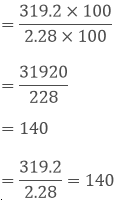(iv) 40.32 by 9.6

= 40.32/9.6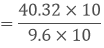= 403.2/96

= 4.2

= 403.2/96 = 4.2

(v) 0.765 by 0.9

= 0.765/0.9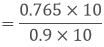= 7.65/9

= 0.85

= 7.65/0.9 = 0.85

(vi) 0.768 by 1.6

= 0.768/1.6= 7.68/16

= 0.48

Question: 5

Divide:
(i) 16.64 by 20
(ii) 0.192 by 12
(iii) 163.44 by 24
(iv) 403.2 by 96
(v) 16.344 by 12
(vi) 31.92 by 228

Solution:

(i) 16.64 by 20

= 16.64/20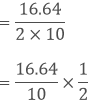= 1.664/2

= 0.832

(ii) 0.192 by 12

= 0.192/12

= 0.016

(iii) 163.44 by 24

= 163.44/24

= 6.81

(iv) 403.2 by 96

= 403.2/96

= 4.2

(v) 16.344 by 12

= 16.344/12

= 1.362

(vi) 31.92 by 228

= 31.92/228

= 0.14

Question: 6

Divide:

(i) 15.68 by 20
(ii) 164.6 by 200
(iii) 403.80 by 30

Solution:

(i) 15.68 by 20

=15.68/20=1.568/2

= 0.784

(ii) 164.6 by 200

Question: 7

(i) 76 by 0.019

(ii) 88 by 0.08

(iii) 148 by 0.074

(iv) 7 by 0.014

(iii) 403.80 by 30

Solution:

(i) 76 by 0.019

= 76/0.019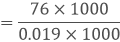= 76000/19

= 4000

(ii) 88 by 0.08

= 88/0.08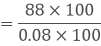= 8800/8

=1100

(iii) 148 by 0.074

= 148/0.074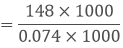= 148000/74

= 2000

(iv) 7 by 0.014

= 70.014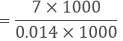= 7000/14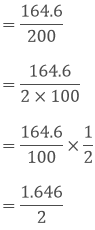= 0.823

(iii) 403.80 by 30

= 403.80/30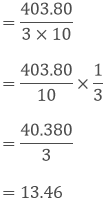Question: 8

Divide:

(i) 20 by 50

(ii) 8 by 100

(iii) 72 by 576

(iv) 144 by 15

Solution:

(i) 20 by 50
= 20/50
= 0.4
(ii) 8 by 100
= 8/100
By shifting the decimal point to the left
= 8/100
= 0.08
(iii) 72 by 576
= 72/576
= 0.125

(iv)144 by 15
= 144/15

= 9.6

Question: 9

A vehicle covers a distance of 43.2 km in 2.4 litres of petrol. How much distance will it travel in 1 litre of petrol?

Solution:

Distance covered in 2.4 litres of petrol = 43.2 km

Distance covered in 1 litre of petrol = 43.2/2.4

= 18km

The distance travelled in 1 litre of petrol is 18 km

Question: 10

The total weight of some bags of wheat is 1743 kg. If each bag weights 49.8 kg, how many bags are there?

Solution:

Total weight of bags of wheat = 1743 kg

Each bag weight = 49.8 kg

No of bags = 1743/49.8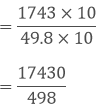= 35

Therefore the total numbers of bags are 35

Question: 11

Shikha cuts 50 m of cloth into pieces of 1.25 m each. How many pieces does she get?

Solution:

Total length of cloth = 50 m

Length of each piece of cloth = 1.25 m

Number of pieces = 50/1.25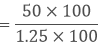= 5000/125

= 40 pieces

Therefore Shikha got 40 pieces

Question: 12

Each side of a rectangular polygon is 2.5 cm in length. The perimeter of the polygon is 12.5 cm. How many sides does the polygon have?

Solution:

Length of each side of rectangular polygon = 5.2 cm
Perimeter of polygon = 12.5 cm
No of sides polygon has = 12.5 cm
No of sides polygon have = 12.5/2.5= 5

Therefore the sides of the polygon is 5

Question: 13

The product of two decimals is 42.987. If one of them is 12.46, find the other.

Solution:

We have,

The product of the given decimals = 42.987

one decimal = 12.46

The other decimal = 42.987/12.46

= 3.45

The number is 3.45

Question: 14

The weight of 34 bags of sugar is 3483.3 kg. If all bags weigh equally, find the weight of each bag.

Solution:

Total weight of sugar = 3483.3kg

No of bags = 34

Weight of each bag = 3483.3/34

= 102.45 kg

Therefore weight of each bag is 102.45 kg

Question: 15

How many buckets of equal capacity can be filled from 586.5 litres of water, if each bucket has capacity of 8.5 litres?

Solution:

Capacity of each bucket = 805 litres

Total water available = 586.5 litres

Number of buckets = 805/586.5= 69

Total number of buckets is 69
```### Course Features

• 728 Video Lectures
• Revision Notes
• Previous Year Papers
• Mind Map
• Study Planner
• NCERT Solutions
• Discussion Forum
• Test paper with Video Solution